# Motion

The two bodies move in the same direction evenly in a straight line, at speeds of 5 cm/s and 10 cm/s. The movement of the first body started 2 seconds earlier than the movement of the second body, from a point located at a distance of 20 cm from the starting point (where the second body was located). Draw a suitable chart in which you can find the place and time of the meeting.

s =  26.6667 cm
t =  4.6667 s

### Step-by-step explanation:

s = 4(t+2)
s = 10t-20

s = 4•(t+2)
s = 10•t-20

s-4t = 8
s-10t = -20

s = 80/3 ≈ 26.666667
t = 14/3 ≈ 4.666667

Our linear equations calculator calculates it.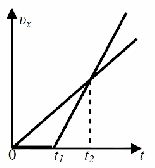Did you find an error or inaccuracy? Feel free to write us. Thank you!Tips to related online calculators
Do you have a system of equations and looking for calculator system of linear equations?
Do you want to convert length units?
Do you want to convert velocity (speed) units?
Do you want to convert time units like minutes to seconds?

## Related math problems and questions:

• CollisionThe two bodies, whose initial distance is 240 m, move evenly against each other consistently. The first body has an initial velocity of 4 m/s and an acceleration of 3 m/s2, the second body has an initial speed of 6 m/s and an acceleration of 2 m/s2. Fin
• Motion 2At 10 o'clock started from place A car speed at 90 km/h. 20 minutes after started from the place B at speed 60 km/h another car. Distance places A and B is 120 kilometers. What time and where they meet?
• Two shipsThe distance from A to B is 300km. At 7 am started from A to B a ferry with speed higher by 20 km/h than a ship that leaves at 8 o'clock from B to A. Both met at 10h 24min. Determine how far they will meet from A and when they reach the destination.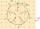John can ran around a circular track in 20 seconds and Eddie in 30 seconds. Two seconds after Eddie starts, John starts from the same place in opposite direction. When will they meet?
• The tourist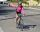The tourist came started from the hostel at an average speed of 5km/h. Half an hour later, the bicyclist started along the same route at a speed of 20km/h. How many minutes will a cyclist catch up and how many kilometers will he go?
• Up and down motionWe throw the body from a height h = 5 m above the Earth vertically upwards v0 = 10 m/s. How long before we have to let the second body fall freely from the same height to hit the Earth at the same time?
• Against each other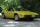From two points A, B distant 23 km at the same time started two cars against each other at speeds 41 km/h and 65 km/h. How long does cars meet and what distance passes each of them?
• Two cyclists 2At the same time, two cyclists left the towns A and B at constant speeds. The first one going from town A to town B, and the second one from town B to town A. At one point of the trip they met. After they met, the first cyclist arrived at town B in 36min,
• An accelerationThe car goes on a straight road at a speed of 72 km/h. At some point, the driver starts to brake and stops the car in 5 seconds. Find: (a) the acceleration during braking (b) the distance traveled during braking.
• Cars 2Opava is 360 km distance from Prešov. At 8:00 hour, two cars started against each other from this cities at average speeds 88 km/h and 121 km/h. What hour will it meet and what distance it's travel if the car started from Prešov is faster?
• Sebastian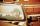From Kovalov Sebastian walks at 6 km / h started at 8:00 in the direction of Kuty. From Kuty, the godfather is driving at 50 km/h and started at 8:30. The distance is 24 km. When and where grandfather will take Sebastian to the car.
• Škoda carsAt 8:30 am, Škoda 120 started from Prievidza at 60 km/h in the direction of Bratislava. At 9:00 started from Prievidza at the same direction Škoda Rapid at 90 km/h. Where and when will Škoda Rapid catch up Skoda 120?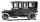Car started at 11:40 at speed 54 km/h from A and to B arrived at 12:20. Determine the distance A and B.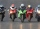On a 2,550 m long circuit, two motorcycles go at such speeds that they meet every minute if they go against each other and run every 5 minutes if they go in the same direction. Find their speeds.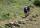From the cottage started first group of tourists at 7:00 AM at speed 4 km/h. The second started after them 21 minutes later at speed 6 km/h. How long and how many kilometers from the cottage will catch the first group?The free fall body has gone 10m in the last 0.5s. Find the body speed at the moment of impact.The distance between cities A and B is 132 km. At 9.00 am, the cyclist started the bike at an average speed of 24 km/h, and at 10.00 am started from the B cyclist at an average speed of 30 km/h. How long and far from A will they both meet?